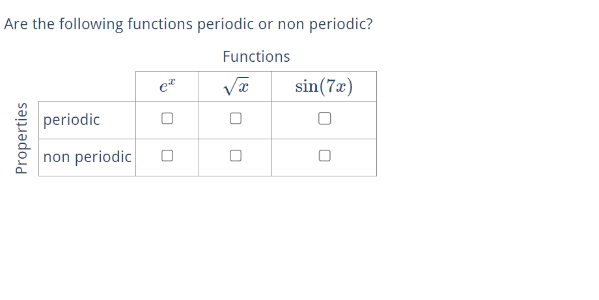Index

Working with MUMIE as author

• Initial steps:
• Articles:
• Problems:
• Programming with Python
• New Visualizations with JSXGraph
• Old Visualizations:
• Media Documents:

Working with MUMIE as teacher

Using MUMIE via plugin in local LMS

FAQ for examination lecturers

Overview

Go back to the old wiki

We revise and update this wiki. We apologize for the inconvenience this may cause you.

There are two levels for setting the type of answers in a question: at the question level, with a uniform type for all answers in the question, or within each answer environment. Hence a question can for instance wrap an answer of type Multiple Choice and an other one of typy Input Fields.

There are Multiple Choice problems of different types: unique, multiple, yes-no, .... For details see the corresponding paragraph below. Do not confuse the term "type of MC-problems" with the term "type of answers".

Setting the type is done by the \type{...}-command, and the position of the command determines
how it works.

Example 1 (one type for all answers fields):

123456789101112\begin{question}    \type{input.number} % set for all answers     \begin{answer}        ...    \end{answer}     \begin{answer}        ...    \end{answer} \end{question} 

Example 2 (possibly different types for each answer):

12345678910111213141516171819\begin{question}    \type{input.generic} % optional      \begin{answer}        \type{input.number} % this answer has to be a number        ...    \end{answer}     \begin{answer}        \type{input.function} % this answer is a function        ...    \end{answer}     \begin{answer}        \type{mc.multiple} % this answer block consists of a MC-question.        ...    \end{answer} \end{question} 

In the second example, the command \type{input.generic} can be omitted, but each answer
environment needs to have a \type{...}-command. It is not possible to set a specific type
on question level and just overwrite it for some answers.

Notice that some features like [\permuteAnswers](input.fields.md#permutable-answers) won't be available if a question has different types of answers.

## input-types

More detailed information on input field questions can be found under the following link:
Overview input field questions

Here is just an overview of the types:

Type Preview
input.number

example and detailed explanation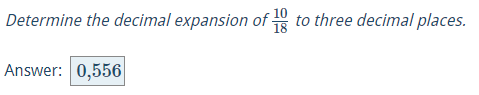input.finite-number-set

example

By default only numbers (e.g. 1, 5, 1.2, 2/3) are accepted values for the user input. With the command \allowForInput the set of valid symbols for the input can be extended. That allows for example to ask for multiple of pi: \allowForInput{+ - * / pi}.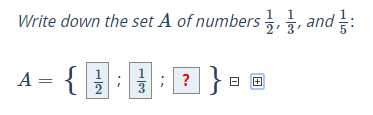input.function
with the pre-given solution or subject to given conditions

example and detailed explanation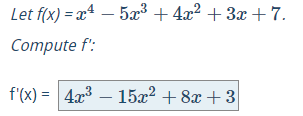input.cases.function
Designed for case differentiations of e.g. absolute value functions.
Note that you can only use this input type in an answer environment.

example and detailed explanation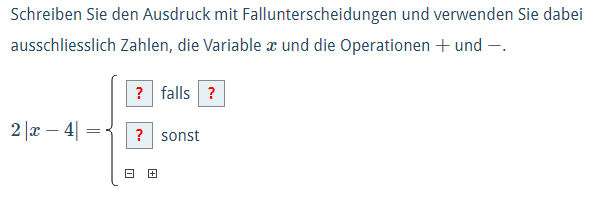input.interval
By default only numbers (e.g. 1, 5, 1.2, 2/3) are accepted values for the user input. With the command \allowForInput the set of valid symbols for the input can be extended. That allows for example to ask for multiple of pi: \allowForInput{+ - * / pi}.

example and detailed explanation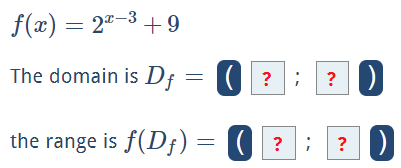input.matrix
If the solution to a question is a row vector, a column vector or a matrix,
the generic problem is of the type input.matrix.

example (cross product) and detailed explanation

Use checkAsFunction for comparing matrices with (multivariate) functions as matrix entries.

example (Jacobian matrix)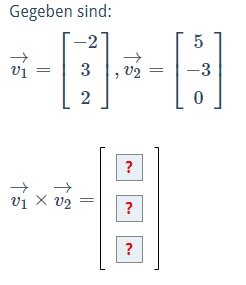input.text

examples and detailed explanation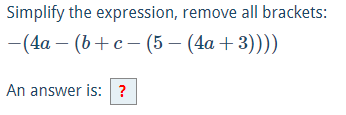input.truth-table
The boolean values can be changed by mouse click

example and detailed explanation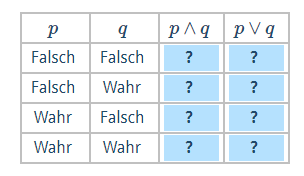## Multiple Choice types

More detailed information on MC questions can be found under the following link:
Multiple choice questions

Here is just an overview of the types:

Type Preview
mc.multiple
Multiple choice questions

example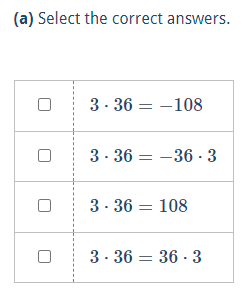mc.unique
Multiple choice questions

example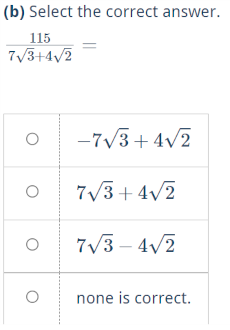mc.yesno
Multiple choice questions

example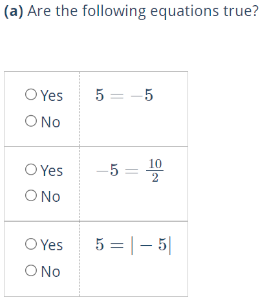mc.matrix
Multiple choice questions

example or detailed explanations